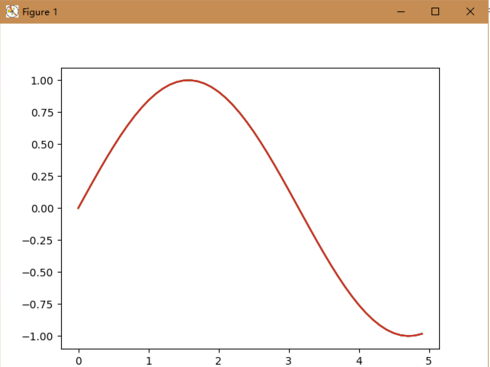# 怎样用python函数画图像• 爱喝马黛茶的安东尼   2019-10-11 11:52:013877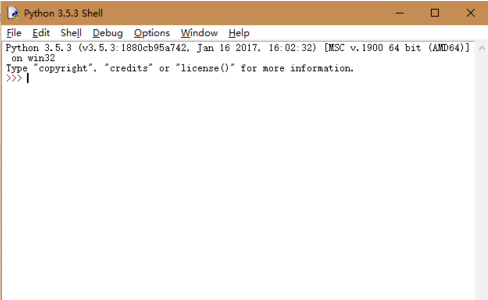```>>> import numpy as np
>>> import matplotlib.pyplot as plt```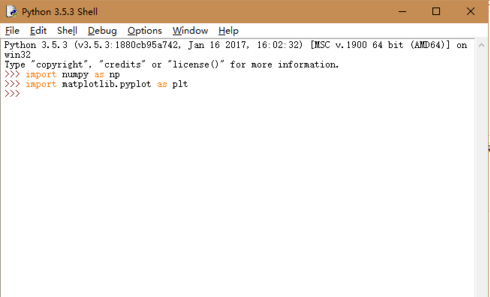```>>> x=np.arange(0,5,0.1);
>>> y=np.sin(x);```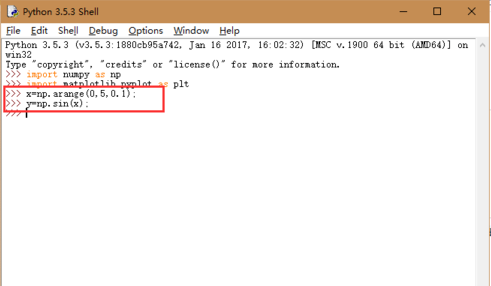`>>>plt.plot(x,y)`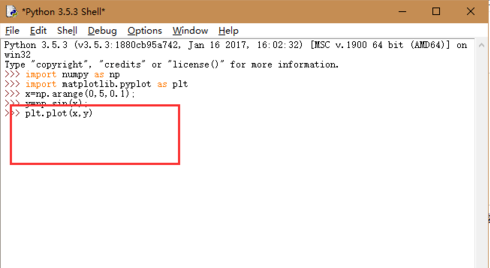`>>>plt.show()`